Like   Tweet   in# Jacksonville History Matters: NEWS & EVENTS

 table div table+table+table+table div table{width:100%;padding:0}table div table+table+table+table div table img{width:96.23%;padding:0;float:none}table div table+table+table+table div table td{width:100%;padding:0 1.88% 18px}/* styles */## Speaking of Jacksonville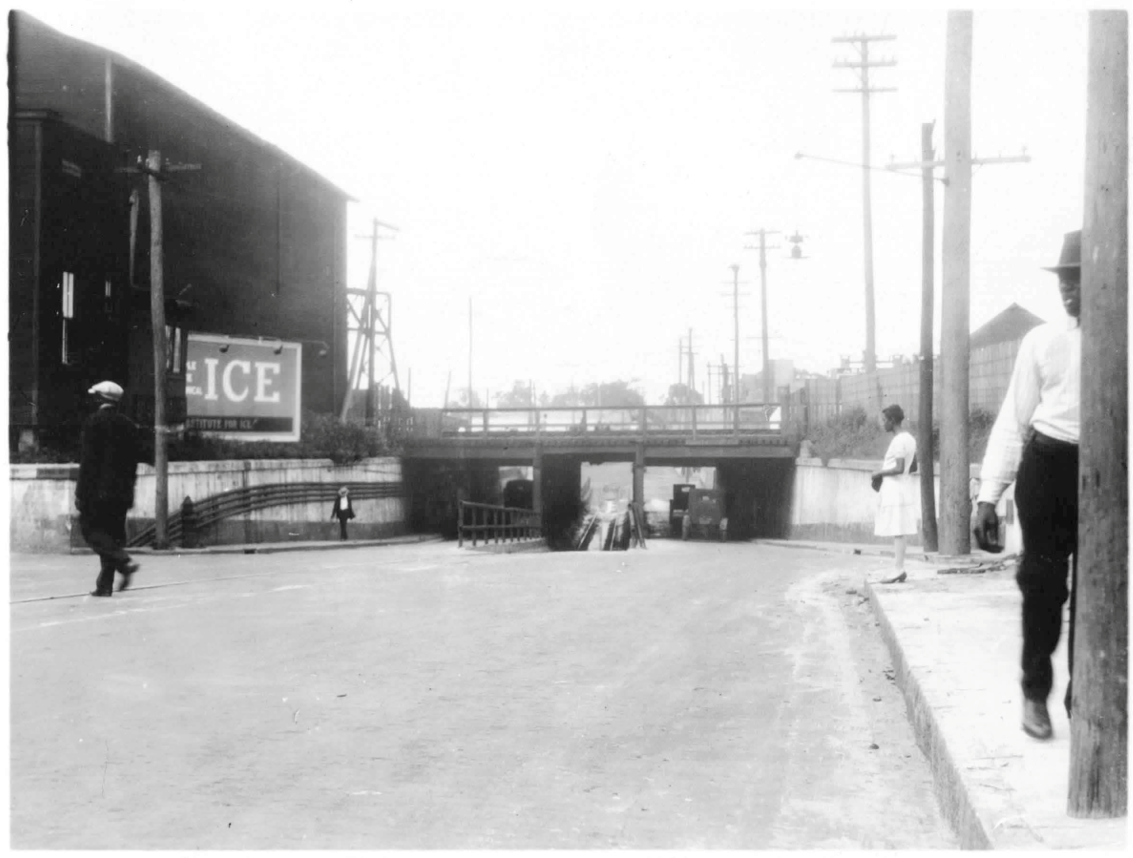Simons called this the Myrtle Avenue subway, a constricted, flood-prone bottleneck where the street passed beneath the railroad tracks just west of the downtown Union Station terminal. His recommendations for improvements or replacement of this feature, already obsolete in 1929, apparently fell on deaf ears. Credit: UNF Carpenter Library, Special CollectionsYes, it’s still the old the Myrtle Avenue subway, photographed by Alan Bliss on July 19, 2014. Truck traffic must be diverted several blocks to the west, owing to the low (9 foot) vertical clearance beneath the rail-bed. Above Myrtle Avenue here is Interstate 95. Credit: Alan Bliss
 table div table+table+table+table+table+table+table+table div table{width:100%;padding:0}table div table+table+table+table+table+table+table+table div table img{width:96.23%;padding:0;float:none}table div table+table+table+table+table+table+table+table div table td{width:100%;padding:0 1.88% 18px}/* styles */## Stories from the Gullah Geechee Community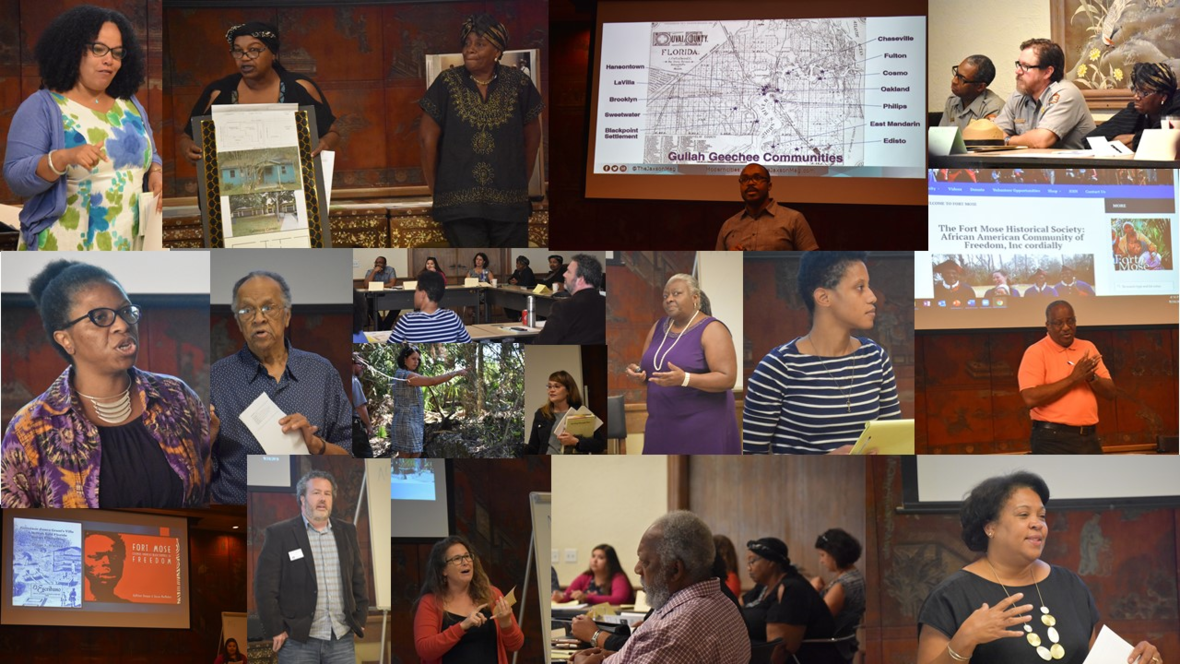/* styles */ Earlier this month, the Jacksonville Historical Society had the honor of attending a stakeholders meeting with the Gullah Geechee Cultural Heritage Corridor Commission. This full day event was held at White Oak Conservation in Yulee, and included a lunch and tour of the property between morning and afternoon sessions. The goal was to better understand what is being done in Nassau, Duval and St. Johns counties to help preserve, protect and share the history and culture of the Gullah Geechee communities of coastal Florida. Oftentimes, these stories are hiding in plain sight.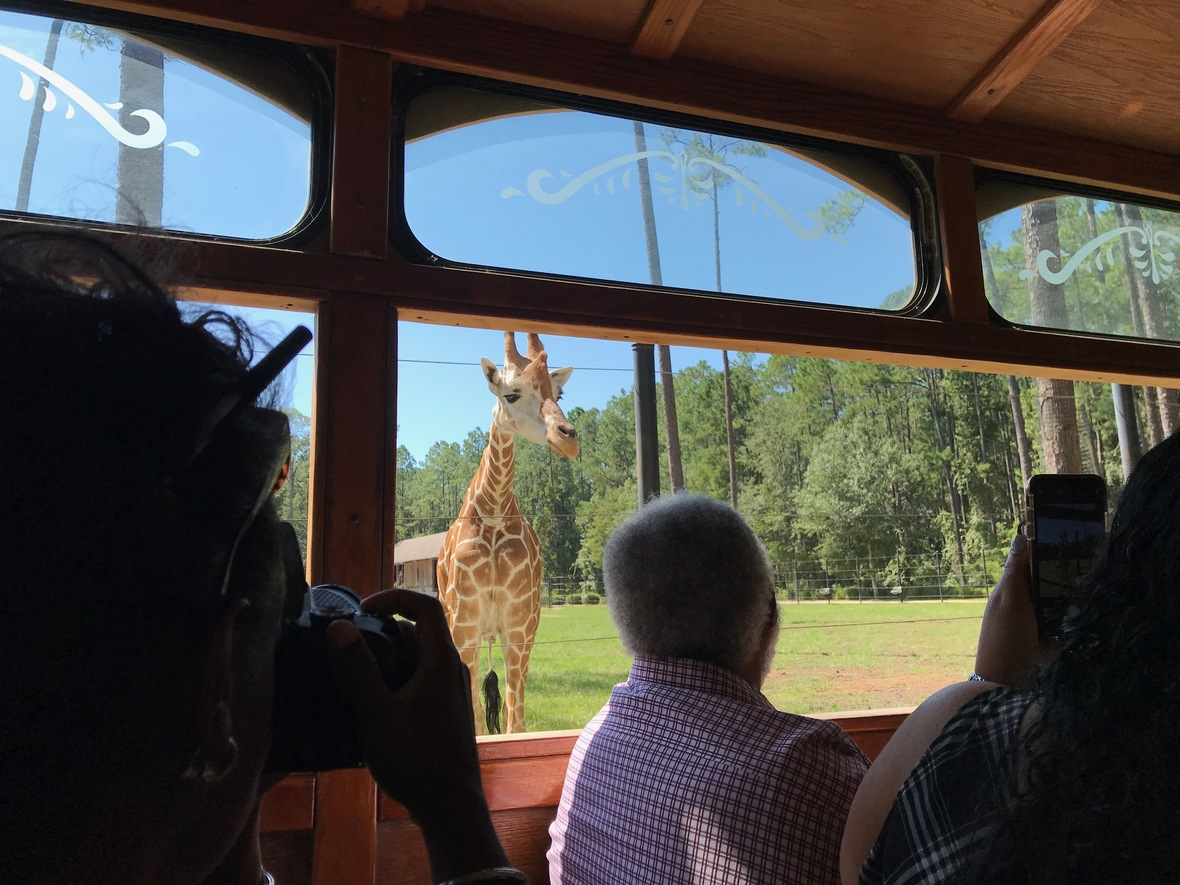A resident of White Oak pays us a visit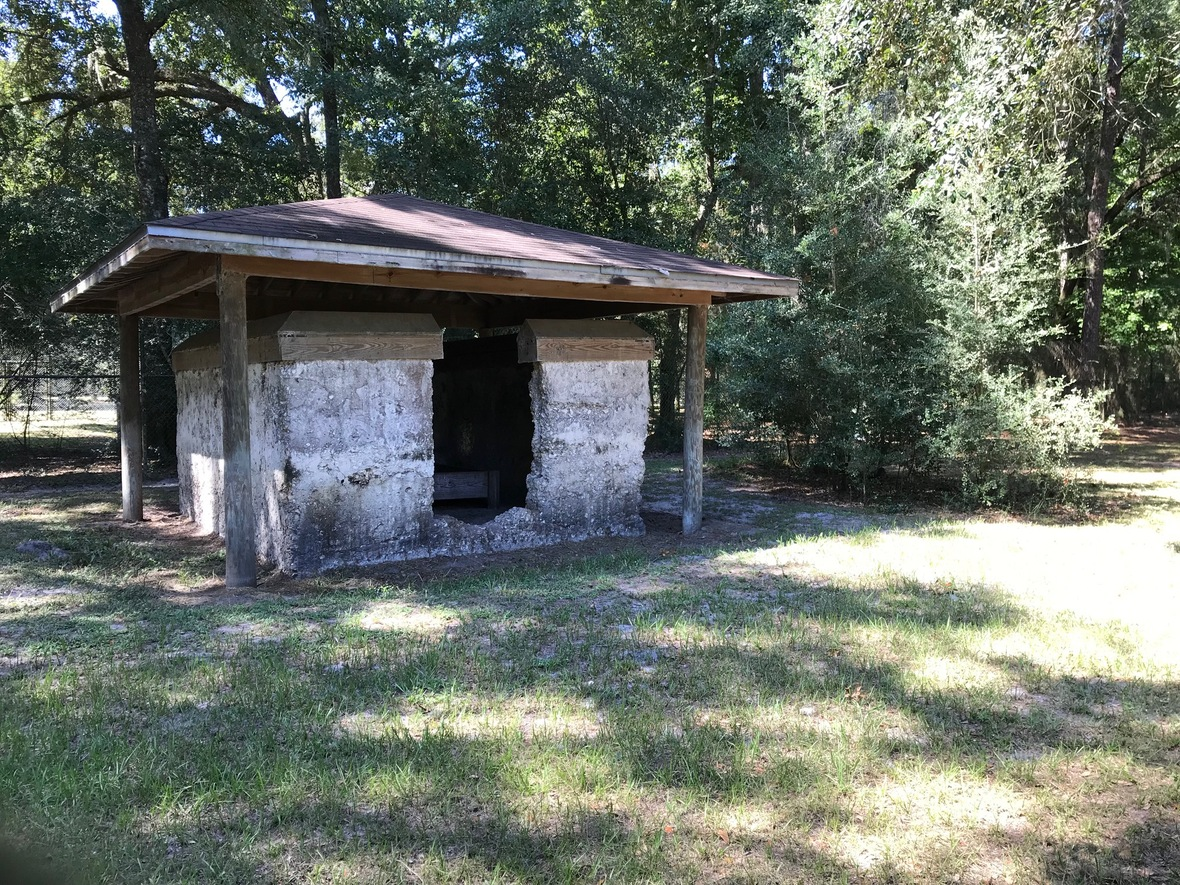A rare glimpse of the days when enslaved people toiled over this land
 /* styles */ With an emphasis on the question: What do we know about Gullah Geechee Heritage?, each of us presented our goals to highlight these seldom told stories. One way the JHS can do this is to digitize its oral history collection, Conversations From Cosmo, compiled by Lynn Corley in 2015. This collection has already been transcribed, but remains on micro cassette. In order to preserve the voices of one of our very own Gullah communities, we'll need to digitize them as soon as possible. We are grateful to the Gullah Geechee Cultural Heritage Corridor Commission for giving us a place at the table. We all have a responsibility to preserve these incredible stories and ensure that they are not lost to the ravages of time. Mitchell Hemann Head Archivist
 table div table+table+table+table+table+table+table+table+table+table+table+table+table+table div table{width:100%;padding:0}table div table+table+table+table+table+table+table+table+table+table+table+table+table+table div table img{width:96.23%;padding:0;float:none}table div table+table+table+table+table+table+table+table+table+table+table+table+table+table div table td{width:100%;padding:0 1.88% 18px}/* styles */## In Memoriam: Marjorie Broward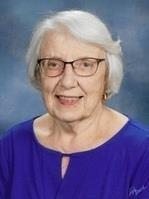It is with a heavy heart that we announce the passing of Marjorie Ann Grimes Broward, who died on September 11th, 2019. A member and long time supporter of the Jacksonville Historical Society, Mrs. Broward led an impressive life.

Born on July 28th, 1924 in Grand Meadow MN, she earned her graduate library degree at the University of Michigan in 1948. She went on to establish an Architecture and City Planning Library at Georgia Tech. It was there that she met Robert C. Broward, an architecture student and protégé of Frank Lloyd Wright. They were soon married in 1952 and moved to Jacksonville shortly thereafter where Mr. Broward started his practice in 1953. Marjorie began working with local libraries and started a bookmobile for underserved areas of the city. The Browards divorced in 1963, and Marjorie and her daughter Kristanna moved to Boulder, CO, where she established a business library at the University of Colorado. She then spent many years consulting and teaching in Australia. Later, she travelled the world computerizing theological seminary libraries in places like Malaysia, Namibia, Jamaica, Nairobi, Kenya and India. Returning to Jacksonville to be near her grandchildren, Mrs. Broward helped to start JAX READS in 2002. Though divorced, Marjorie and Robert Broward remained close, and she was instrumental in helping him write his book The Broward Family: From France to Florida, 1764-2011, published in 2011 by the Jacksonville Historical Society.

We've recently gotten to know Mrs. Broward quite well, when she generously donated a large collection of personal and research material belonging to Robert Broward. She was a friend and supporter of the JHS, and we always looked forward to seeing her face in the audience at our programs. She was a remarkable woman, and we wish peace to her and to all who loved and admired her.

 table div table+table+table+table+table+table+table+table+table+table+table+table+table+table+table+table+table div table{width:100%;padding:0}table div table+table+table+table+table+table+table+table+table+table+table+table+table+table+table+table+table div table img{width:96.23%;padding:0;float:none}table div table+table+table+table+table+table+table+table+table+table+table+table+table+table+table+table+table div table td{width:100%;padding:0 1.88% 18px}/* styles */## Edible Design-Building Opportunity!

 /* styles */ CALLING ALL BUILDERS! Reservations for Gingerbread entries are now being accepted. If you or your group wish to participate, please send in your registration soon. Reservations will be accepted until space is full. The Gingerbread Extravaganza is one of the most creative design shows that occurs in Northeast Florida each year. Amateurs and professionals of all skill levels design and construct 85% edible creations based on a subject or theme of their interest. The building experience reinforces team-building and project management skills. Participation provides great PR exposure as the event receives significant media coverage and over 4,000 visitors parade through the show each year. A completed Builder Permit Form is required to reserve space in the show. Click on Gingerbread Builder link below to reserve your spot. Space is limited.
 table div table+table+table+table+table+table+table+table+table+table+table+table+table+table+table+table+table+table+table+table+table div table td,table.module-20{width:100%;padding:0}table div table+table+table+table+table+table+table+table+table+table+table+table+table+table+table+table+table+table+table+table+table div table{width:100%;float:none;margin-left:auto;margin-right:auto;padding:0}table div table+table+table+table+table+table+table+table+table+table+table+table+table+table+table+table+table+table+table+table+table div table a{border:0 none;text-decoration:none}table div table+table+table+table+table+table+table+table+table+table+table+table+table+table+table+table+table+table+table+table+table div table img{width:100%!important;border:0 none;text-decoration:none}/* styles */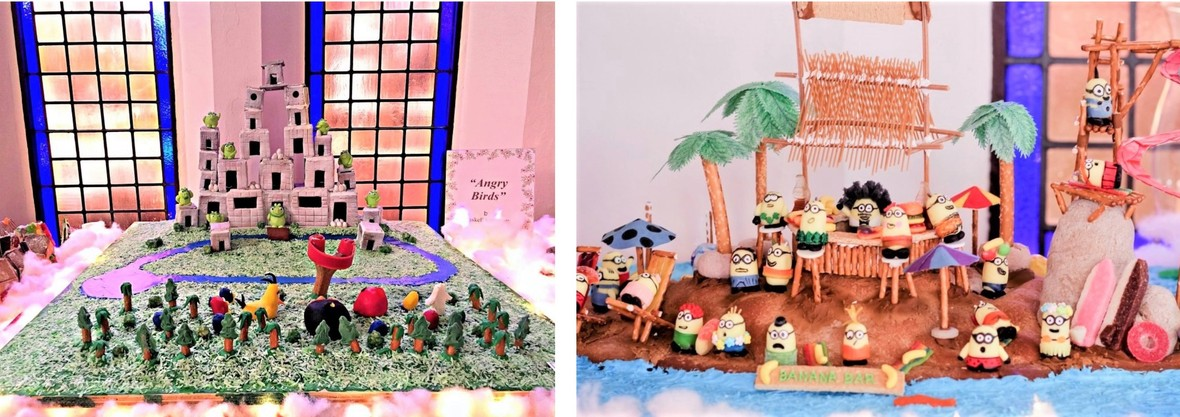Edible creations from years gone by
 table div table+table+table+table+table+table+table+table+table+table+table+table+table+table+table+table+table+table+table+table+table+table+table div table{width:100%;padding:0}table div table+table+table+table+table+table+table+table+table+table+table+table+table+table+table+table+table+table+table+table+table+table+table div table img{width:96.23%;padding:0;float:none}table div table+table+table+table+table+table+table+table+table+table+table+table+table+table+table+table+table+table+table+table+table+table+table div table td{width:100%;padding:0 1.88% 18px}/* styles */## Merrill House Docent Training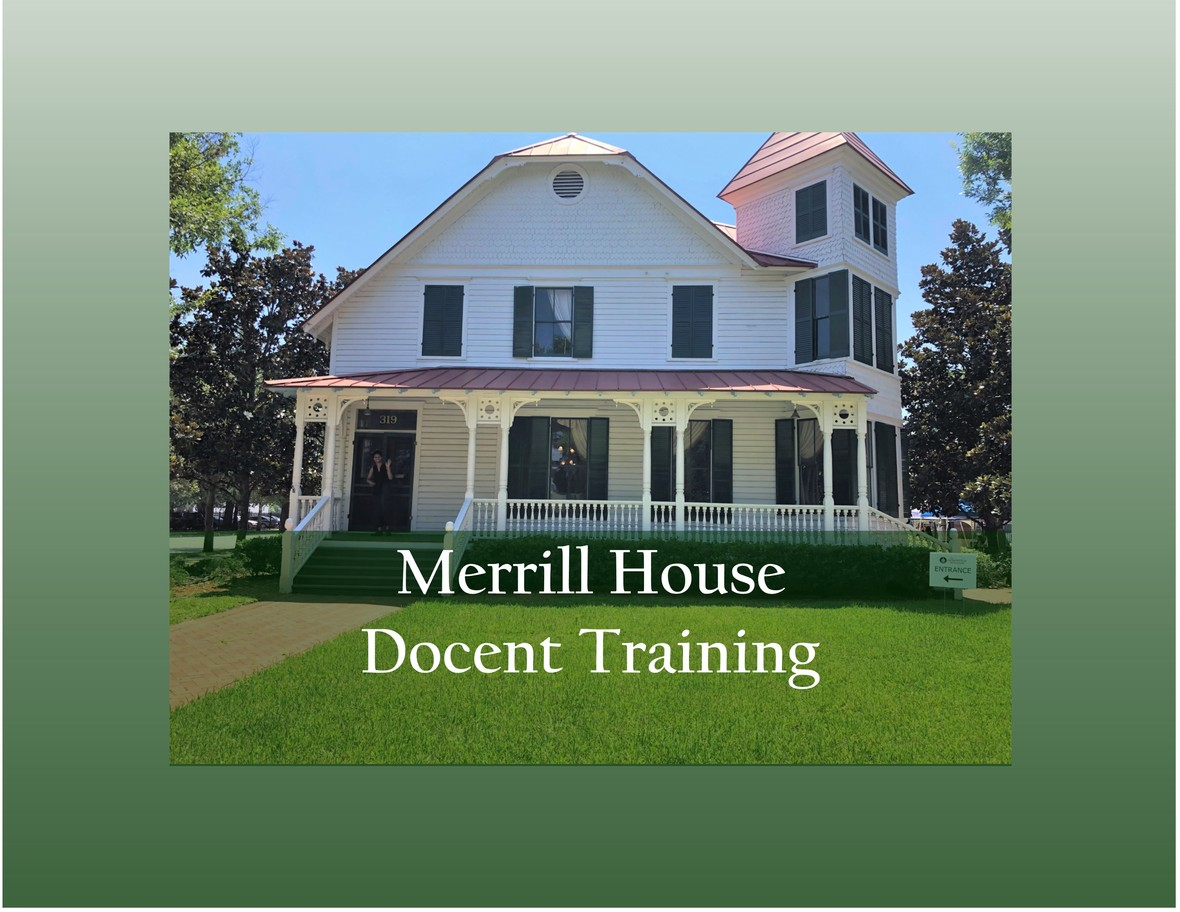FALL DOCENT TRAINING SCHEDULE

Year round you can find Merrill House Docents lead small group tours of this famous Jacksonville home. Through guided tours, we share early twentieth century lifestyles with visitors. Many visitors are expected this December during the 16th Annual Gingerbread Extravaganza. Expected docent time commitment is a few hours per week, based on your availability. If you can join us in this important role, please RSVP to attend one of the Docent Training sessions:

# RSVP - DOCENT TRAINING: Monday, Oct. 14, 9am

 table div table+table+table+table+table+table+table+table+table+table+table+table+table+table+table+table+table+table+table+table+table+table+table+table+table+table div table{width:100%;padding:0}table div table+table+table+table+table+table+table+table+table+table+table+table+table+table+table+table+table+table+table+table+table+table+table+table+table+table div table img{width:96.23%;padding:0;float:none}table div table+table+table+table+table+table+table+table+table+table+table+table+table+table+table+table+table+table+table+table+table+table+table+table+table+table div table td{width:100%;padding:0 1.88% 18px}/* styles */## Holiday Parties at Old St. Andrews!

 table.module-27{width:75.47%;padding:0}table div table+table+table+table+table+table+table+table+table+table+table+table+table+table+table+table+table+table+table+table+table+table+table+table+table+table+table+table div table{width:75.47%;float:none;margin-left:auto;margin-right:auto;padding:0}table div table+table+table+table+table+table+table+table+table+table+table+table+table+table+table+table+table+table+table+table+table+table+table+table+table+table+table+table div table a{border:0 none;text-decoration:none}table div table+table+table+table+table+table+table+table+table+table+table+table+table+table+table+table+table+table+table+table+table+table+table+table+table+table+table+table div table img{width:100%!important;border:0 none;text-decoration:none}table div table+table+table+table+table+table+table+table+table+table+table+table+table+table+table+table+table+table+table+table+table+table+table+table+table+table+table+table div table td{width:100%;padding:0}/* styles */
 /* styles */ Special opportunity: Holiday parties at Old St. Andrews! Impress your friends, clients or staff by hosting your holiday party in this beautiful building surrounded by Gingerbread Extravaganza. Our Venue Coordinators have catering resources available to provide a turnkey event for you! For more information, contact our Venue Coordinators: Jade Stanley: 904-878-8122 Rob Jackson: 904-878-2241 Email: Events@JaxHistory.org
 table div table+table+table+table+table+table+table+table+table+table+table+table+table+table+table+table+table+table+table+table+table+table+table+table+table+table+table+table+table+table div table{width:100%;padding:0}table div table+table+table+table+table+table+table+table+table+table+table+table+table+table+table+table+table+table+table+table+table+table+table+table+table+table+table+table+table+table div table img{width:96.23%;padding:0;float:none}table div table+table+table+table+table+table+table+table+table+table+table+table+table+table+table+table+table+table+table+table+table+table+table+table+table+table+table+table+table+table div table td{width:100%;padding:0 1.88% 18px}/* styles */## Upcoming Events

 table div table+table+table+table+table+table+table+table+table+table+table+table+table+table+table+table+table+table+table+table+table+table+table+table+table+table+table+table+table+table+table+table div table td,table.module-31{width:100%;padding:0}table div table+table+table+table+table+table+table+table+table+table+table+table+table+table+table+table+table+table+table+table+table+table+table+table+table+table+table+table+table+table+table+table div table{width:100%;float:none;margin-left:auto;margin-right:auto;padding:0}table div table+table+table+table+table+table+table+table+table+table+table+table+table+table+table+table+table+table+table+table+table+table+table+table+table+table+table+table+table+table+table+table div table a{border:0 none;text-decoration:none}table div table+table+table+table+table+table+table+table+table+table+table+table+table+table+table+table+table+table+table+table+table+table+table+table+table+table+table+table+table+table+table+table div table img{width:100%!important;border:0 none;text-decoration:none}/* styles */

# Visit www.jaxhistory.org to make reservations for these and other events.

 table div table+table+table+table+table+table+table+table+table+table+table+table+table+table+table+table+table+table+table+table+table+table+table+table+table+table+table+table+table+table+table+table+table+table div table{width:100%;padding:0}table div table+table+table+table+table+table+table+table+table+table+table+table+table+table+table+table+table+table+table+table+table+table+table+table+table+table+table+table+table+table+table+table+table+table div table img{width:96.23%;padding:0;float:none}table div table+table+table+table+table+table+table+table+table+table+table+table+table+table+table+table+table+table+table+table+table+table+table+table+table+table+table+table+table+table+table+table+table+table div table td{width:100%;padding:0 1.88% 18px}/* styles */## Accepting Gently Used Holiday Treasures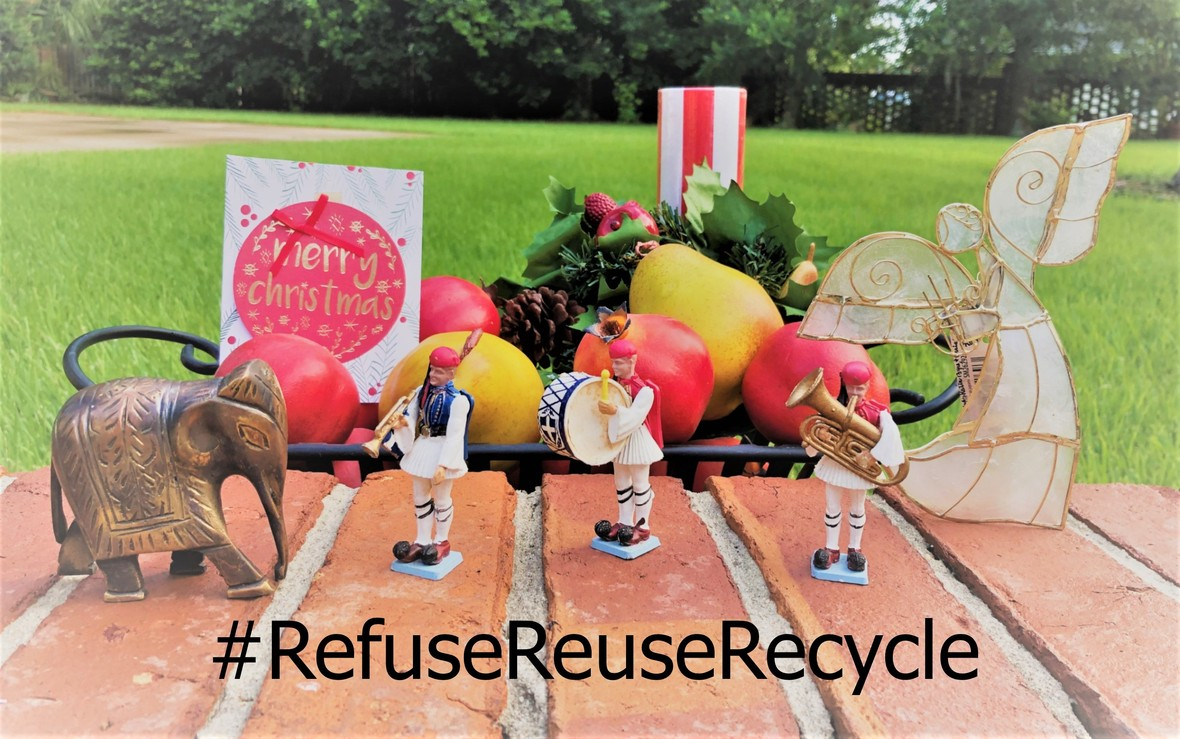JHS is accepting gently used holiday treasures to re-sell in the Gingerbread Holiday Shop. Your donations will find new homes and help generate operating funds for JHS. Bring them to any and all JHS events, or drop them off at the office between 9am - 5pm.

 table div table+table+table+table+table+table+table+table+table+table+table+table+table+table+table+table+table+table+table+table+table+table+table+table+table+table+table+table+table+table+table+table+table+table+table+table+table div table{width:100%;padding:0}table div table+table+table+table+table+table+table+table+table+table+table+table+table+table+table+table+table+table+table+table+table+table+table+table+table+table+table+table+table+table+table+table+table+table+table+table+table div table img{width:96.23%;padding:0;float:none}table div table+table+table+table+table+table+table+table+table+table+table+table+table+table+table+table+table+table+table+table+table+table+table+table+table+table+table+table+table+table+table+table+table+table+table+table+table div table td{width:100%;padding:0 1.88% 18px}/* styles */## Holiday Gift Giving with Meaning

 /* styles */ Make gift giving easy this year. Order some of these impressive books or historic ornaments for your friends and family. JHS is proud to be a retailer for the 50th Anniversary Edition of the Quiet Revolution, which chronicles the consolidation of Jacksonville and Duval County governments. Hardback history books are always an impressive gift to recieve. And you will find many more great ideas in the JHS Bookstore!
 table div table+table+table+table+table+table+table+table+table+table+table+table+table+table+table+table+table+table+table+table+table+table+table+table+table+table+table+table+table+table+table+table+table+table+table+table+table+table+table+table div table td,table.module-39{width:100%;padding:0}table div table+table+table+table+table+table+table+table+table+table+table+table+table+table+table+table+table+table+table+table+table+table+table+table+table+table+table+table+table+table+table+table+table+table+table+table+table+table+table+table div table{width:100%;float:none;margin-left:auto;margin-right:auto;padding:0}table div table+table+table+table+table+table+table+table+table+table+table+table+table+table+table+table+table+table+table+table+table+table+table+table+table+table+table+table+table+table+table+table+table+table+table+table+table+table+table+table div table a{border:0 none;text-decoration:none}table div table+table+table+table+table+table+table+table+table+table+table+table+table+table+table+table+table+table+table+table+table+table+table+table+table+table+table+table+table+table+table+table+table+table+table+table+table+table+table+table div table img{width:100%!important;border:0 none;text-decoration:none}/* styles */
 table.module-41{width:99.81%;padding:0}table div table+table+table+table+table+table+table+table+table+table+table+table+table+table+table+table+table+table+table+table+table+table+table+table+table+table+table+table+table+table+table+table+table+table+table+table+table+table+table+table+table+table div table{width:99.81%;float:none;margin-left:auto;margin-right:auto;padding:0}table div table+table+table+table+table+table+table+table+table+table+table+table+table+table+table+table+table+table+table+table+table+table+table+table+table+table+table+table+table+table+table+table+table+table+table+table+table+table+table+table+table+table div table a{border:0 none;text-decoration:none}table div table+table+table+table+table+table+table+table+table+table+table+table+table+table+table+table+table+table+table+table+table+table+table+table+table+table+table+table+table+table+table+table+table+table+table+table+table+table+table+table+table+table div table img{width:100%!important;border:0 none;text-decoration:none}table div table+table+table+table+table+table+table+table+table+table+table+table+table+table+table+table+table+table+table+table+table+table+table+table+table+table+table+table+table+table+table+table+table+table+table+table+table+table+table+table+table+table div table td{width:100%;padding:0}/* styles */
 table div table+table+table+table+table+table+table+table+table+table+table+table+table+table+table+table+table+table+table+table+table+table+table+table+table+table+table+table+table+table+table+table+table+table+table+table+table+table+table+table+table+table+table div table{width:100%;padding:0}table div table+table+table+table+table+table+table+table+table+table+table+table+table+table+table+table+table+table+table+table+table+table+table+table+table+table+table+table+table+table+table+table+table+table+table+table+table+table+table+table+table+table+table div table img{width:96.23%;padding:0;float:none}table div table+table+table+table+table+table+table+table+table+table+table+table+table+table+table+table+table+table+table+table+table+table+table+table+table+table+table+table+table+table+table+table+table+table+table+table+table+table+table+table+table+table+table div table td{width:100%;padding:0 1.88% 18px}/* styles */## Our Mission:

To educate and inspire the greater Jacksonville community to value its history, by fostering understanding of how the region's past shapes our present.## JHS is thankful for the many organization that support us in our mission!

 table div table+table+table+table+table+table+table+table+table+table+table+table+table+table+table+table+table+table+table+table+table+table+table+table+table+table+table+table+table+table+table+table+table+table+table+table+table+table+table+table+table+table+table+table+table+table+table div table{width:100%;padding:0}table div table+table+table+table+table+table+table+table+table+table+table+table+table+table+table+table+table+table+table+table+table+table+table+table+table+table+table+table+table+table+table+table+table+table+table+table+table+table+table+table+table+table+table+table+table+table+table div table img{width:96.23%;padding:0;float:none}table div table+table+table+table+table+table+table+table+table+table+table+table+table+table+table+table+table+table+table+table+table+table+table+table+table+table+table+table+table+table+table+table+table+table+table+table+table+table+table+table+table+table+table+table+table+table+table div table td{width:100%;padding:0 1.88% 18px}/* styles */## Staff:

Alan Bliss, Ph.D. , Executive Director | Mitch Hemann, Archivist | Susan Prattos, Administrator | Imani Phillips, Archives & Office Assistant | Sherrard Ceglia, Archives Assistant | Anna Verney, Archives Assistant | David Woodard, Facilities Manager